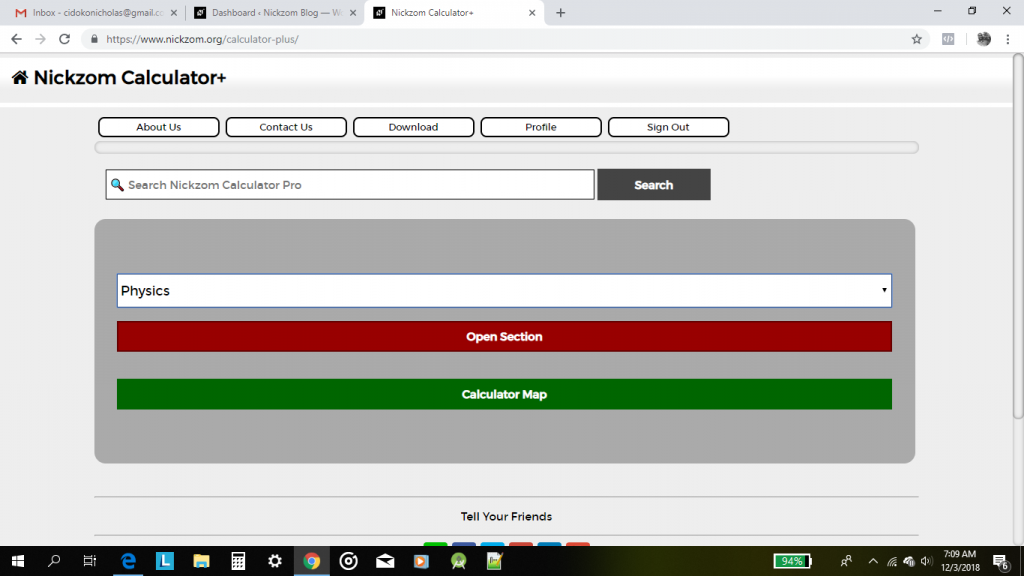## Nickzom Calculator Calculates Range and Maximum Range of a Projectile in Physics

To get the answer for the range of a projectile, there are three essential parameters to be given which are:

• Acceleration due to Gravity
• Initial Velocity
• Angle of Projection

Where as to get the answer for maximum range of a projectile requires two parameters to be given which are:

• Acceleration due to Gravity
• Initial Velocity

Let’s first, proceed to the home page of the app. Select Physics and click on Open SectionThen proceed to click on Projectile## Nickzom Calculator Calculates Maximum Height of a Projectile

According to SoftSchools.com,

A projectile is an object that is given an initial velocity, and is acted on by gravity. The maximum height of the object is the highest vertical position along its trajectory. The maximum height of the projectile depends on the initial velocity v0, the launch angle θ, and the acceleration due to gravity. The unit of maximum height is meters (m).

Nickzom Calculator calculates the maximum height of a projectile given the accurate values for the required parameters. The required parameters to get the answer to maximum height are:

• Acceleration due to Gravity
• Initial Velocity
• Angle of Projection

Let’s get started, first and foremost, proceed to the home page of the app, select Physics and click on Open Section.